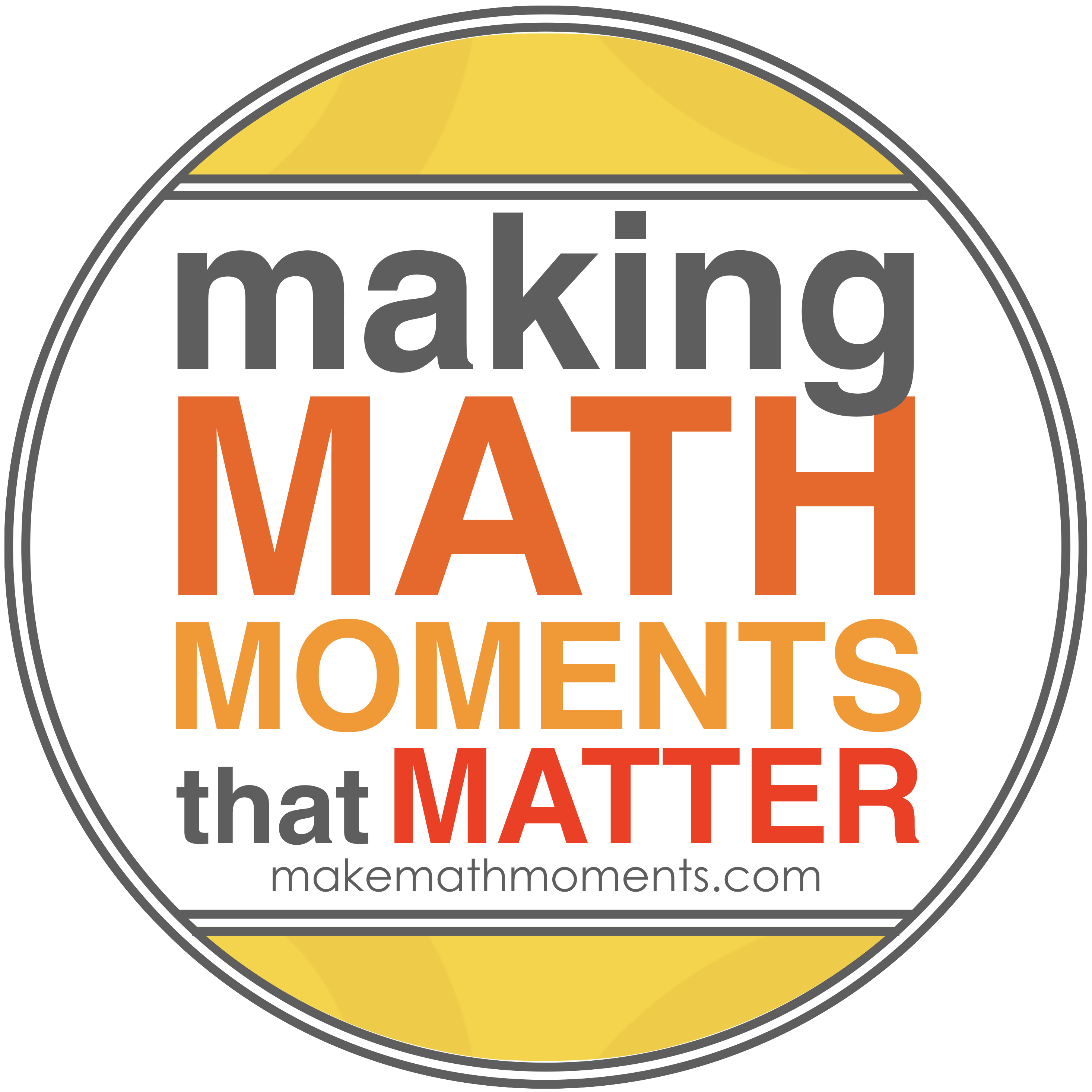# MHF4U

Course Description:

This course extends students’ experience with functions.  Students will investigate the properties of polynomial, rational, logarithmic, and trigonometric functions; broaden their understanding of rates of change; and develop facility in applying these concepts and skills.  Students will also refine their use of the mathematical processes necessary for success in senior mathematics.  This course is intended both for students who plan to study mathematics in university and for those wishing to consolidate their understanding of mathematics before proceeding to any one of a variety of univerisity programs.

Overall Learning Expectations:

By the end of this course, students will:

• demonstrate an understanding of the relationship between exponential expressions and logarithmic expressions, evaluate logarithms, and apply the laws of logarithms to simpligy numeric expressions
• identify and describe some key features of the graphs of logarithmic functions, make connections between the numeric, graphical, and algebraic representations of logarithmic functions, and solve related problems graphically
• solve exponential and simple logarithmic equations in one variable algebraically, including those arising from real-world applications
• demonstrate an understanding of the meaning and application of radian measure
• make connections between trigonometric ratios and the graphical and algebraic representations of the corresponding trigonometric functions and between trigonometric functions and their reciprocals, and use these connections to solve problems
• solve problems involving trigonometric equations and prove trigonometric identities
• identify and describe some key features of polynomial functions, and make connections between numerical, graphical, and algebraic representation of polynomial functions
• identify and describe some key features of the graphs of rational functions, and represent rational functions graphically
• solve problems involving polynomial and simple rational equations graphically and algebraically
• demonstrate an understanding of solving polynomial and simple rational inequalities
• demonstrate an understanding of average and instantaneous rate of change, and determine, numerically and graphically, and interpret the average rate of change of a function over a given interval and the instantaneous rate of change of a function at a given point

• determine functions that result from the addition, subtraction, multiplication, and division of two functions and from the composition of two functions, describe some properties of the resulting functions, and solve related problems
• compare the characteristics of functions, and solve problems by modelling and reasoning with functions, including problems with solutions that are not accessible by standard algebraic techniques

Evaluation:

Term Work                                                                                         70%

Knowledge / Understanding                              30 %

Inquiry / Thinking                                              20 %

Communication                                                 20 %

Application / Making Connections                     30 %

Assessment activities during the course will be comprised of a variety of methods and strategies.  (eg.  Assignments, projects, tests, journals, performances, conferences, daily work, etc.)

Final Evaluation                                                                                 30%

The final evaluation will consist of, but is not limited to, a final examination, written during the examination schedule.

Classroom Rules:

Students are expected to follow these rules so that mathematics education can be successful.

a) BE ON TIME

b) COME PREPARED FOR CLASS

c) PARTICIPATE IN CLASS – Keep good notes while the lesson is being taught, pay

d) RESPECT THE RIGHTS OF OTHERS – Work quietly and co-operate so as not to

disturb other students who are trying to learn.

e) CATCH UP ON ANY WORK MISSED – When returning from an absence, catch up

on missed work as quickly as possible.  This is your responsibility.

f) COMPLETE ANY HOMEWORK ASSIGNED – If work is not completed in class,

complete it before the next class so that you may benefit from the discussions based on

the homework.

g) REMAIN IN YOUR DESK until the teacher dismisses the class.

h) FOLLOW ESTABLISHED SCHOOL ROUTINES AND BEHAVIOUR CODES – see

student handbook.

Learning Materials Required:

• Textbook
• Notebook – 3 ring binder with paper
• Pencil, eraser, ruler
• Scientific calculator
• Student Handbook